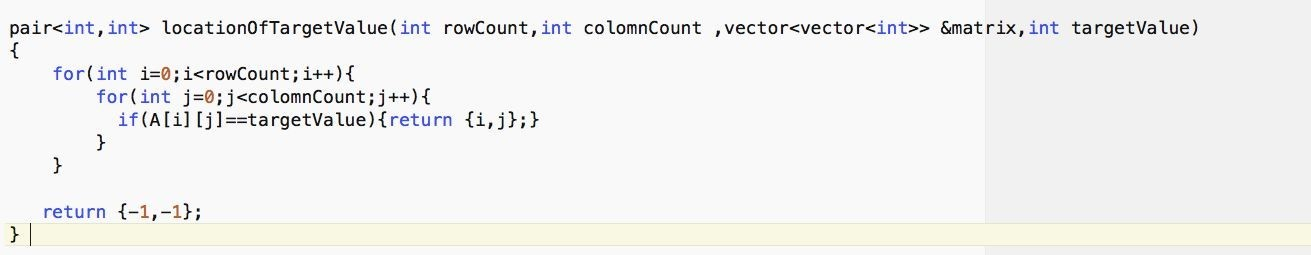## Search in a row-wise and column-wise sorted matrix

Aim - Given a row-wise and column-wise sorted array with N rows and M columns and an integer x return the row and column index such that matrix[row][col] = x, if x not found return {-1,-1} .

Ex- matrix = -3 1 31 40

10 33 40 660

22 43 161 702

x=22

Ans will be {2,0} i.e. row - 2 and col - 0 (0 based indexing).

# Naive’s Approach-

Method- Simply check every element of the array and if it matches with x return row and column.Time complexity- O(n*m) - As we are simply iterating over each element of n*m array.

Can We do better?

We have not used a condition which is given to us that is every row and columns are sorted. Let’s utilize this to improve our time complexity to O(m+n).

# Efficient Solution-

Approach:​ The simple idea is to remove a row or column in each comparison until we find the required result. Start searching from the top-right corner of the array as towards left, numbers will decrease and toward down, numbers will increase.

Ex- ​-3 1 31 40

10 33 40 660

22 43 161 702

28 44 164 800

Starting from 40, its left contains(same row) all smaller number whereas it’s down(same column) contain all larger number so if the required value is greater than 40 we ignore row for further search and if require value is less than 40 we ignore column and if it is 40 than we simply return its x,y coordinates. So in each comparison, we are able to reduce our search space by 1 row or 1 column. So in the worst case, we can have O(m+n) comparisons. Let’s write a blueprint of our approach-

There are 3 cases at each point -

1. ​ The required number is greater than the current number: ​ We can ignore that column means can shift from {x,y}->{x,y-1}.

2. ​ The required number is smaller than the current number: ​ We can ignore that row means can shift from {x,y}->{x+1,y}.

3.​The required number is equal to the current number: ​ Simply return this pair of {x,y}.

If at any moment x=-1 or y=-1 then we can see that no solution exists and our answer is

{-1,-1}

# Implementation :

Finally achieved O(m+n) time complexity, Auxiliary Space- O(1)